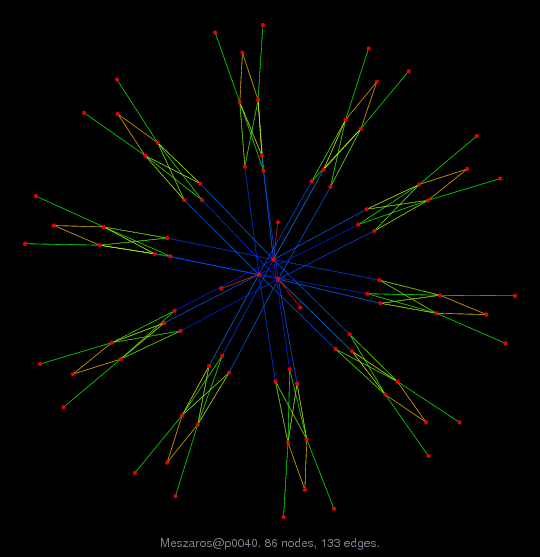Matrix: Meszaros/p0040

Description: linear programming problem, C. Meszaros test set(bipartite graph drawing)• Matrix group: Meszaros
• download as a MATLAB mat-file, file size: 1 KB. Use UFget(1742) or UFget('Meszaros/p0040') in MATLAB.

 Matrix properties number of rows 23 number of columns 63 nonzeros 133 structural full rank? yes structural rank 23 # of blocks from dmperm 1 # strongly connected comp. 1 explicit zero entries 0 nonzero pattern symmetry 0% numeric value symmetry 0% type integer structure rectangular Cholesky candidate? no positive definite? no

 author editor C. Meszaros date 2004 kind linear programming problem 2D/3D problem? no

 Additional fields size and type b full 23-by-1 c full 63-by-1 lo full 63-by-1 hi full 63-by-1 z0 full 1-by-1

Notes:

```http://www.sztaki.hu/~meszaros/public_ftp/lptestset
Converted to standard form via Resende and Veiga's mpsrd:
minimize c'*x, subject to A*x=b and lo <= x <= hi
```

 Ordering statistics: result nnz(V) for QR, upper bound nnz(L) for LU, with COLAMD 355 nnz(R) for QR, upper bound nnz(U) for LU, with COLAMD 100

 SVD-based statistics: norm(A) 6027.47 min(svd(A)) 1 cond(A) 6027.47 rank(A) 23 sprank(A)-rank(A) 0 null space dimension 0 full numerical rank? yes

 singular values (MAT file): click here SVD method used: s = svd (full (R)) ; where [~,R,E] = spqr (A') with droptol of zero status: ok